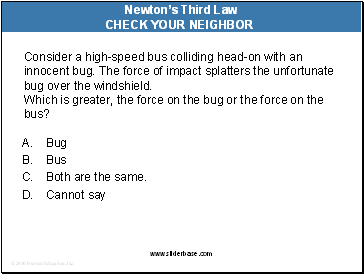# Newton’s third law of motionPage 2

#### WATCH ALL SLIDES

A. a slight air resistance.

nonexistent in this case.

you pulling Earth upward.

None of the above.

Newton’s Third Law of Motion

Slide 10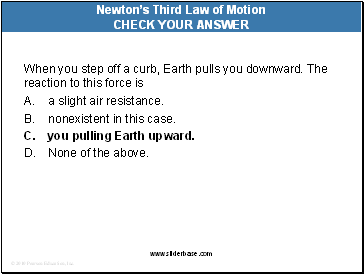When you step off a curb, Earth pulls you downward. The reaction to this force is

A. a slight air resistance.

nonexistent in this case.

you pulling Earth upward.

None of the above.

Newton’s Third Law of Motion

Slide 11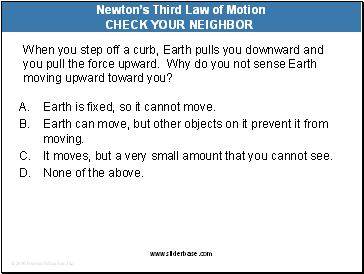When you step off a curb, Earth pulls you downward and you pull the force upward. Why do you not sense Earth moving upward toward you?

A. Earth is fixed, so it cannot move.

Earth can move, but other objects on it prevent it from moving.

It moves, but a very small amount that you cannot see.

None of the above.

Newton’s Third Law of Motion

Slide 12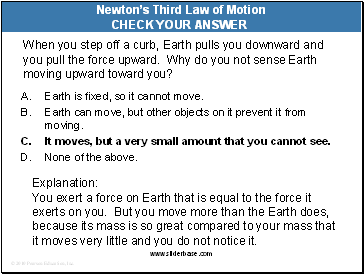When you step off a curb, Earth pulls you downward and you pull the force upward. Why do you not sense Earth moving upward toward you?

A. Earth is fixed, so it cannot move.

Earth can move, but other objects on it prevent it from moving.

It moves, but a very small amount that you cannot see.

None of the above.

Newton’s Third Law of Motion

Explanation:

You exert a force on Earth that is equal to the force it exerts on you. But you move more than the Earth does, because its mass is so great compared to your mass that it moves very little and you do not notice it.

Slide 13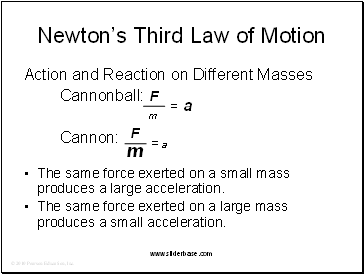Newton’s Third Law of Motion

Action and Reaction on Different Masses

Cannonball:

Cannon:

The same force exerted on a small mass produces a large acceleration.

The same force exerted on a large mass produces a small acceleration.

Slide 14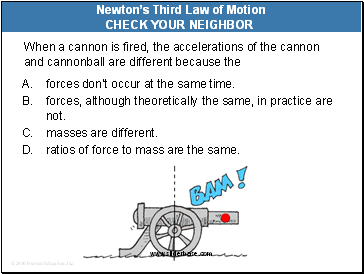When a cannon is fired, the accelerations of the cannon and cannonball are different because the

A. forces don’t occur at the same time.

forces, although theoretically the same, in practice are not.

masses are different.

ratios of force to mass are the same.

Newton’s Third Law of Motion

Slide 15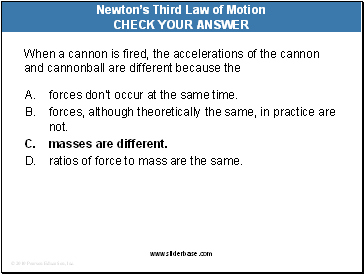When a cannon is fired, the accelerations of the cannon and cannonball are different because the

A. forces don’t occur at the same time.

forces, although theoretically the same, in practice are not.

masses are different.

ratios of force to mass are the same.

Newton’s Third Law of Motion